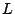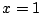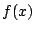# Critical values

The critical value of an-function (the first nonzero Taylor coefficient at the symmetry point of the functional equation) contains significant arithmetic information. The most famous example is the case of an-function associated with an elliptic curve. The Birch and Swinnerton-Dyer conjecture states that the order of vanishing at the critical point gives the rank of the group of rational points on the curve, and the critical value gives a combination of other important arithmetic information about the curve.

Random matrix theory can be used to study critical values by using an analogy between the-function and the characteristic polynomial. In this case the behavior of the-function at the critical point corresponds to the behavior atfor the characteristic polynomial.

At present the most extensive work has been done on the study of ranks of elliptic curves, but it is likely that random matrix methods will soon be employed to study other questions related to critical values.

Back to the main index for L-functions and Random Matrix Theory.